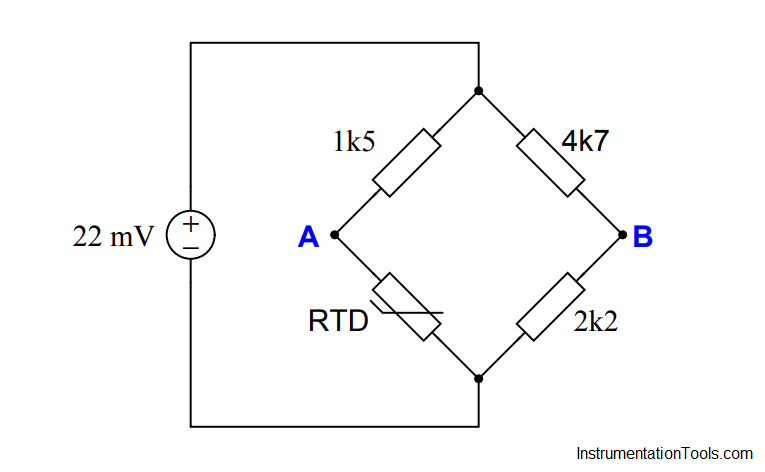# Resistance Temperature Detector (RTD) Questions – 2

Resistance Temperature Detector (RTD) Questions

Calculate the voltage across the bridge (VAB) at the following RTD temperatures, assuming a 604 Ω nickel-iron RTD with an alpha value of 0.00518:• T = 0 oC ; VAB = _____ millivolts
• T = 81 oC ; VAB = _____ millivolts
• T = -45 oC ; VAB = _____ millivolts
• T = 320 oF ; VAB = _____ millivolts